# Profit and Loss - Quantitative Aptitude (MCQ) questions

Dear Readers, Welcome to Quantitative Aptitude Profit and Loss questions and answers with explanation. These Profit and Loss solved examples with shortcuts and tricks will help you learn and practice for your Placement Test and competitive exams like Bank PO, IBPS PO, SBI PO, RRB PO, RBI Assistant, LIC,SSC, MBA - MAT, XAT, CAT, NMAT, UPSC, NET etc.

After practicing these tricky Profit and Loss multiple choice questions, you will be exam ready to deal with any objective type questions.

1)   A person sold a stove for Rs. 423 and incurred a loss of 6%. At what price would it be sold so as to earn a profit of 8%?
- Published on 11 Apr 17

a. Rs. 525
b. Rs. 500
c. Rs. 490
d. Rs. 486
 Answer  Explanation ANSWER: Rs. 486 Explanation: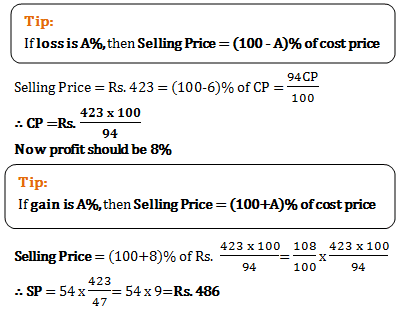2)   A fruit seller buys lemons at 2 for a rupee and sells then at 5 for three rupees. His gain percent is
- Published on 11 Apr 17

a. 10%
b. 15%
c. 20%
d. 25%
 Answer  Explanation ANSWER: 20% Explanation: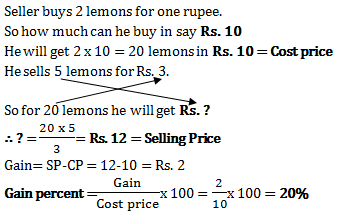3)   A sells a car to B at 10% loss. If B sells it for Rs. 54000 and gains 20%, the cost price of the car for A was
- Published on 11 Apr 17

a. Rs. 25000
b. Rs. 50000
c. Rs. 37500
d. Rs. 60000
 Answer  Explanation ANSWER: Rs. 50000 Explanation: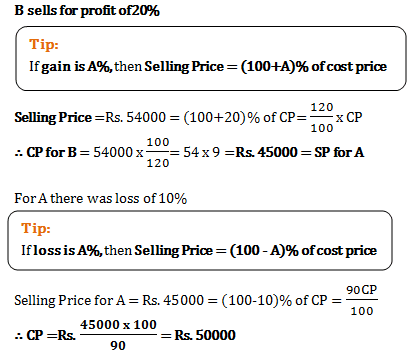4)   Ramesh sold a statue for a price 25% higher than the original price of the statue. He had however bought the statue at 20% discount on the original price. With the profit of Rs. 2025, find the original price of the statue.
- Published on 11 Apr 17

a. Rs. 6000
b. Rs. 7500
c. Rs. 3500
d. Rs. 4500
 Answer  Explanation ANSWER: Rs. 4500 Explanation: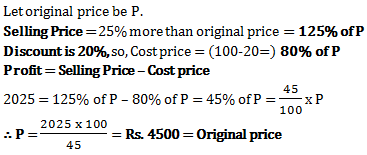5)   If selling price of 40 articles is equal to cost price of 50 articles, the loss or gain percent is
- Published on 05 Apr 17

a. 25% loss
b. 20% loss
c. 25% gain
d. 20% gain
 Answer  Explanation ANSWER: 25% gain Explanation: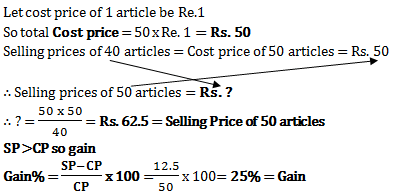6)   Two bicycles were sold for Rs. 3990 each, gaining 5% on one and losing 5% on the other. The gain or loss percent on the whole transaction is
- Published on 05 Apr 17

a. Neither gain nor loss
b. 2.5% gain
c. 2.5% loss
d. 0.25% loss
 Answer  Explanation ANSWER: 0.25% loss Explanation: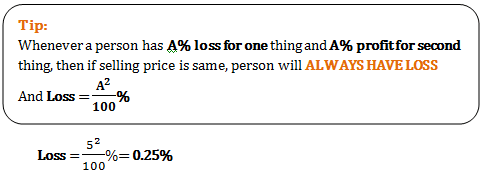7)   The ratio of cost price and selling price is 4:5. The profit percent is
- Published on 05 Apr 17

a. 10%
b. 20%
c. 25%
d. 30%
 Answer  Explanation ANSWER: 25% Explanation: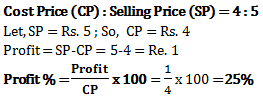8)   If a person sells a ‘sari’ for Rs. 5200, making a profit of 30%, then the cost price of the sari is
- Published on 05 Apr 17

a. Rs. 4420
b. Rs. 4000
c. Rs. 3900
d. Rs. 3800
 Answer  Explanation ANSWER: Rs. 4000 Explanation: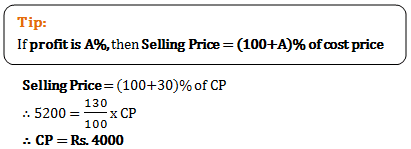9)   A shopkeeper earns a profit of 15% after selling a book at 20% discount on the printed price. The ratio of the cost price and printed price of the book is?
- Published on 05 Apr 17

a. 20:23
b. 23:20
c. 16:23
d. 23:16
 Answer  Explanation ANSWER: 16:23 Explanation: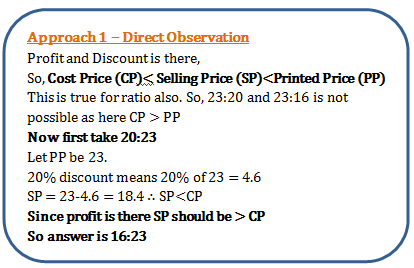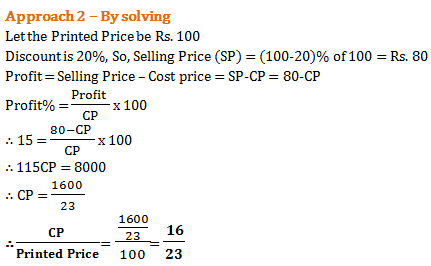10)   Simran bought pet food worth Rs. 56000. She then sold 1/3rd of it incurring a loss of 40%. What profit she must earn on rest of the supplies to nullify this loss?
- Published on 27 Mar 17

a. 25%
b. 20%
c. 45%
d. 50%
 Answer  Explanation ANSWER: 20% Explanation: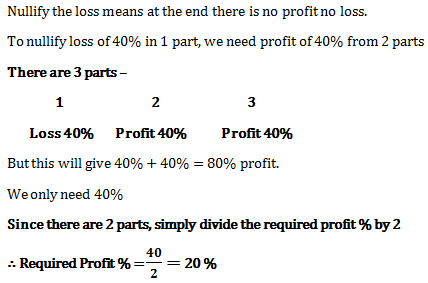1 2 3 4 5 6